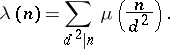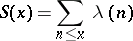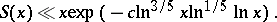# Liouville function

The arithmetic functiondefined bywhereis the number of prime factors of. The Liouville function is closely connected with the Möbius function:In number theory an important estimate is that of the sumas. There is a conjecture thatThe most recent result, obtained by a method of I.M. Vinogradov, has the formThe Liouville function was introduced by J. Liouville.

Liouville function satisfies the explicit formula $$\sum_{n=1}^\infty \frac{\lambda(n)}{\sqrt{n}}g(\log n) = \sum_{\rho}\frac{h( \gamma)\zeta(2 \rho )}{\zeta'( \rho)} + \frac{1}{\zeta (1/2)}\int_{-\infty}^\infty dx \, g(x)$$

where $g(u)= \frac{1}{2\pi} \int_{-\infty}^\infty h(x)\exp(-iux)$ form a Fourier transform pair